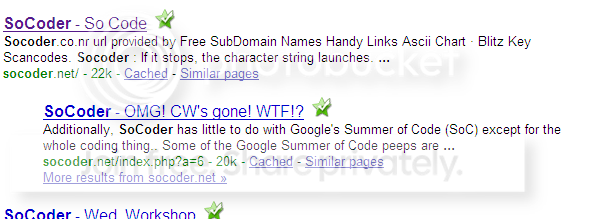-=+=- -=+=- -=+=- -=+=- -=+=- -=+=- -=+=- -=+=- -=+=- -=+=- -=+=- -=+=- -=+=- -=+=- -=+=- -=+=- -=+=- -=+=- -=+=- -=+=- -=+=- -=+=- -=+=- -=+=- -=+=- -=+=- -=+=- -=+=- -=+=- -=+=- (c) WidthPadding Industries 1987 0|679|0 -=+=- -=+=- -=+=- -=+=- -=+=- -=+=- -=+=- -=+=- -=+=- -=+=- -=+=- -=+=- -=+=- -=+=- -=+=- -=+=- -=+=- -=+=- -=+=- -=+=- -=+=- -=+=- -=+=- -=+=- -=+=- -=+=- -=+=- -=+=- -=+=- -=+=- Socoder -> Off Topic -> McAfee SiteAdvisor Posted : Wednesday, 21 May 2008, 12:36 worchyldI recently lauched soccoder with Firefox and the McAfee SiteAdvisor says that this site is bad,When we tested this site we found links to pcriot.com, which we found to be a distributor of downloads some people consider adware, spyware or other potentially unwanted programs.REF: https://www.siteadvisor.com/sites/socoder.net?ref=safe&aff_id=0 Posted : Wednesday, 21 May 2008, 12:48 HoboBenYeah, we had a thread about this. SoCoder is clean, so is the site that it's linked to that's causing the red rating. Basically their rating is the same as saying "right, there's a virus on one .co.uk website somewhere, let's mark every website which links to anything on that top level domain as red. Sorted"... Which is kind of wrong, because that's not how TLDs work. -=-=-Posted : Wednesday, 21 May 2008, 13:12 JL235If it helps there are lots of other sites online that McAfee also says is bad, like the TrainLine.com (or at least sections of it). -=-=- PlayMyCode.com - build and play in your browser, Blog, Twitter.Posted : Sunday, 25 May 2008, 10:36 lyonsmy avg link scanner shows the site with a green tick on google-=-=-Posted : Sunday, 25 May 2008, 11:13 JL235I'm pretty sure the Finjan plugin also gives SoCoder a green light. -=-=- PlayMyCode.com - build and play in your browser, Blog, Twitter.Posted : Sunday, 25 May 2008, 14:57 JayenkaiSo, it's just McAfee being a git, then! I can live with that.. it's pretty much standard-=-=- ''Load, Next List!''